# Degree (mathematics) facts for kids

Kids Encyclopedia Facts
For the corresponding concept in geometry, see Degree (angle).

The degree of a polynomial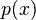$p(x)$, represented by the symbol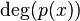$\deg (p(x))$, is the highest exponent that occurs inside that polynomial.

For example, if we look at the polynomial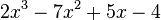$2x^3 - 7x^2 + 5x - 4$, then we can see that the degree of this polynomial is 3, because the highest power in the variable x is 3. It occurs in the term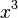$x^3$.

The name of the variable is not important. For example, the polynomial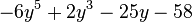$-6y^5 + 2y^3 - 25y - 58$ has degree 5, because the highest power of the variable, in this case y, is 5, which occurs in the term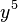$y^5$.

## Related pagesDegree (mathematics) Facts for Kids. Kiddle Encyclopedia.